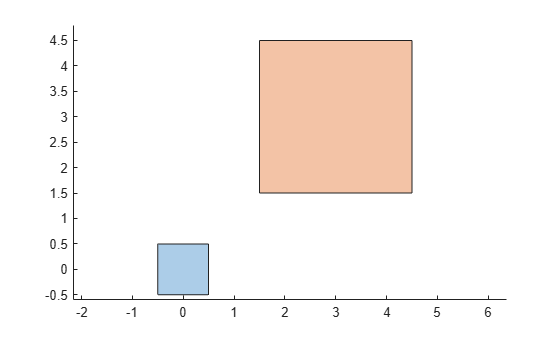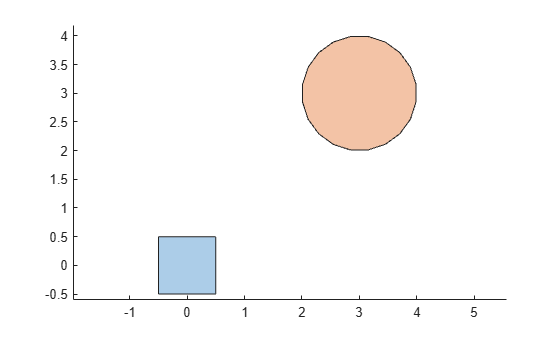# turningdist

Compute turning distance between `polyshape` objects

## Syntax

``td = turningdist(poly1,poly2)``
``td = turningdist(polyvec)``

## Description

````td = turningdist(poly1,poly2)` returns an array of turning distances between the corresponding element pairs of two `polyshape` arrays with compatible sizes. The turning distance between two `polyshape` objects is a measure of how closely their shapes match, regardless of rotation or scaling. A turning distance close to 0 indicates a near match. The larger the value, the more the two shapes differ.TD(i,j) is the turning distance between the ith `polyshape` in `poly1` and the jth `polyshape` in `poly2`.```

example

````td = turningdist(polyvec)` returns a matrix of turning distances between element pairs of a vector of `polyshape` objects.```

## Examples

collapse all

Create and plot two squares of different sizes and locations, represented as `polyshape` objects `poly1` and `poly2`.

```poly1 = nsidedpoly(4,'SideLength',1); poly2 = nsidedpoly(4,'SideLength',3,'Center',[3 3]); plot(poly1) hold on plot(poly2) axis equal hold off```Because the two squares have the same shape despite their scaling, their turning distance is 0.

`td = turningdist(poly1,poly2)`
```td = 0 ```

Create and plot a third `polyshape`, and compare its turning distance to `poly1`. Since their shapes have more differences than `poly1` and `poly2`, the turning distance is larger.

```poly3 = nsidedpoly(20,'Center',[3 3]); plot(poly1) hold on plot(poly3) axis equal hold off````td = turningdist(poly1,poly3)`
```td = 0.4443 ```

## Input Arguments

collapse all

First input `polyshape`, specified as a scalar, vector, matrix, or multidimensional array.

Second input `polyshape`, specified as a scalar, vector, matrix, or multidimensional array.

`polyshape` vector.

## Output Arguments

collapse all

Turning distance, returned as a scalar, vector, matrix, or multidimensional array whose elements are greater than or equal to 0.

• If you input two `polyshape` arguments, then they must have compatible sizes. For example, if two input `polyshape` vectors have different lengths M and N, then they must have different orientations (one must be a row vector and one must be a column vector). `td` is then M-by-N or N-by-M depending on the orientation of each input vector. For more information on compatible array sizes, see Compatible Array Sizes for Basic Operations.

• If you input a single `polyshape` vector with length N, then `td` is N-by-N.

Data Types: `double`

 Arkin, E.M., Chew, L.P., Huttenlocher, D.P., Kedem, K., and Mitchell, J.S.B. "An efficiently computable metric for comparing polygonal shapes." IEEE Transactions on Pattern Analysis and Machine Intelligence. Vol. 13, Number 3, 1991, pp. 209-16. doi:10.1109/34.75509.